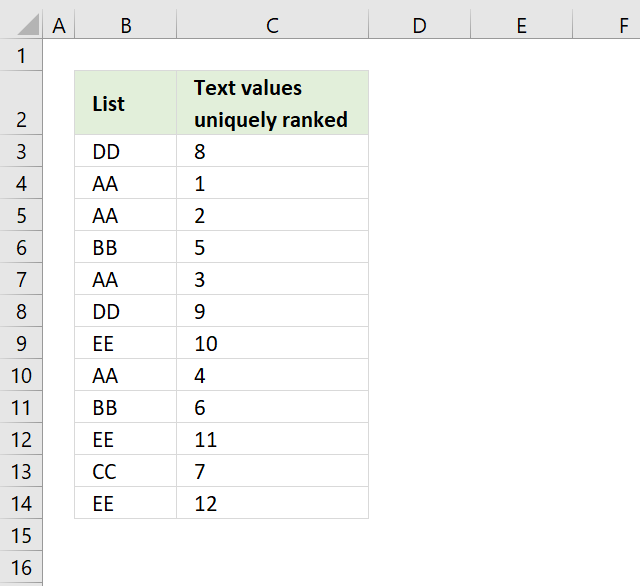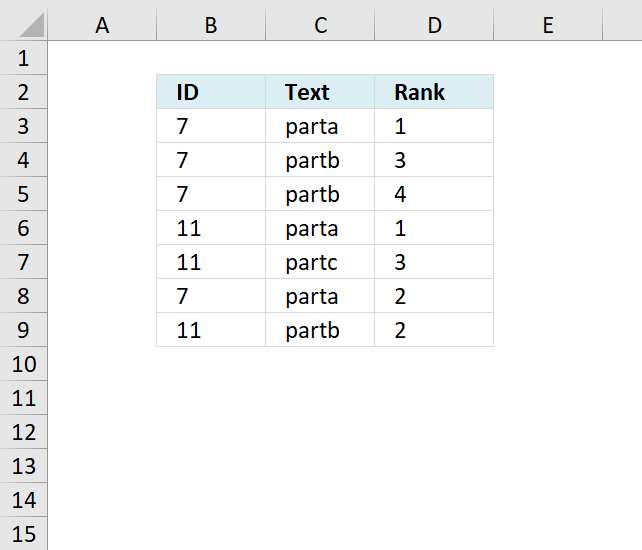Author: Oscar Cronquist Article last updated on March 15, 2022## 1. How to rank text uniquely without duplicatesQuestion: How do I rank text cell values uniquely? If text values were sorted alphabetically from A to Z, the first text value would rank 1 and so on... Duplicate text values rank uniquely.

Formula in cell C3:

=COUNTIF(\$B\$3:\$B\$14,"<"&B3)+COUNTIF(\$B\$3:B3,B3)

Copy cell C3 and paste to cells below as far as needed.

### How does this formula work?

=COUNTIF(\$B\$3:\$B\$14,"<"&B3)+COUNTIF(\$B\$3:B3,B3)

#### Step 1 - Calculate rank

The COUNTIF function is an incredibly versatile function, in this case, instead of counting values based on a condition we simply check if a value is smaller or larger than the others.

Remember, we are using text values so the function returns a rank number based on the position if the list were sorted alphabetically.

COUNTIF(\$B\$3:\$B\$14,"<"&B3)

becomes

=COUNTIF({DD, AA, AA, BB, AA, DD, EE, AA, BB, EE, CC, EE}, "<"&DD)

and returns 7. 7 values are sorted before DD, as if they were sorted from A to Z.

The problem is that value DD has a duplicate and we need to make sure that the duplicate doesn't get the same number as the first instance.

#### Step 2 - Count current value and prior instances of current value

COUNTIF(\$B\$3:B3,B3)

becomes

COUNTIF("DD","DD")

and returns 1.

Note that the cell reference \$B\$3:B3 expands as we copy the cell to cells below.

#### Step 3 - Add values

COUNTIF(\$B\$3:\$B\$14,"<"&B3)+COUNTIF(\$B\$3:B3,B3)

becomes

7 + 1 and returns 8 in cell C3.

#### Final notes

Select B3 and use "evaluate formula" to see how the next cell calculates rank.  Press with left mouse button on "Evaluate" button and excel calculates cell formula step by step.

### Get *.xlsx file

Text values uniquely ranked.xlsx

## 2. How to rank uniquely based on a conditionThe following formula ranks text values in column C uniquely based on the category in column B.

Formula in D3:

=SUMPRODUCT((C3&ROW()>\$C\$3:\$C\$9&ROW(\$C\$3:\$C\$9))*(B3=\$B\$3:\$B\$9))+1

### Explaining formula in cell D3

#### Step 1 - Concatenate cell C3 and current row number

The ROW function returns the row number from a cell reference, if a cell ref is omitted then the row number of the current cell is returned.

The ampersand character & concatenates two values.

C3&ROW()

becomes

"parta"&3

and returns parta3.

#### Step - 2 Concatenate each cell in column C and corresponding row numbers row-wise

\$C\$3:\$C\$9&ROW(\$C\$3:\$C\$9)

becomes

{"parta";"partb";"partb";"parta";"partc";"parta";"partb"}&{3;4;5;6;7;8;9}

and returns

{"parta3";"partb4";"partb5";"parta6";"partc7";"parta8";"partb9"}

#### Step 3 - Compare value with array using the larger than sign

(C3&ROW()>\$C\$3:\$C\$9&ROW(\$C\$3:\$C\$9))

becomes

parta3>{"parta3";"partb4";"partb5";"parta6";"partc7";"parta8";"partb9"}

and returns

{FALSE;FALSE;FALSE;FALSE;FALSE;FALSE;FALSE}.

The number concatenated to each value makes it unique.

#### Step 4 - Compare categories with current cell

(B3=\$B\$3:\$B\$9)

becomes

7={7;7;7;11;11;7;11}

and returns

{TRUE;TRUE;TRUE;FALSE;FALSE;TRUE;FALSE}

#### Step - 5 Multiply arrays

(C3&ROW()>\$C\$3:\$C\$9&ROW(\$C\$3:\$C\$9))*(B3=\$B\$3:\$B\$9)

becomes

{FALSE;FALSE;FALSE;FALSE;FALSE;FALSE;FALSE}*{TRUE;TRUE;TRUE;FALSE;FALSE;TRUE;FALSE}

and returns

{0;0;0;0;0;0;0}

#### Step 6 - Sum values in array

SUMPRODUCT((C3&ROW()>\$C\$3:\$C\$9&ROW(\$C\$3:\$C\$9))*(B3=\$B\$3:\$B\$9))

becomes

SUMPRODUCT({0;0;0;0;0;0;0})

and returns 0.

#### Step 7 - Add 1

SUMPRODUCT((C3&ROW()>\$C\$3:\$C\$9&ROW(\$C\$3:\$C\$9))*(B3=\$B\$3:\$B\$9)) + 1

becomes

0 + 1 equals 1 in cell D3.

Get Excel *.xlsx file

ranking text with condition.xlsx